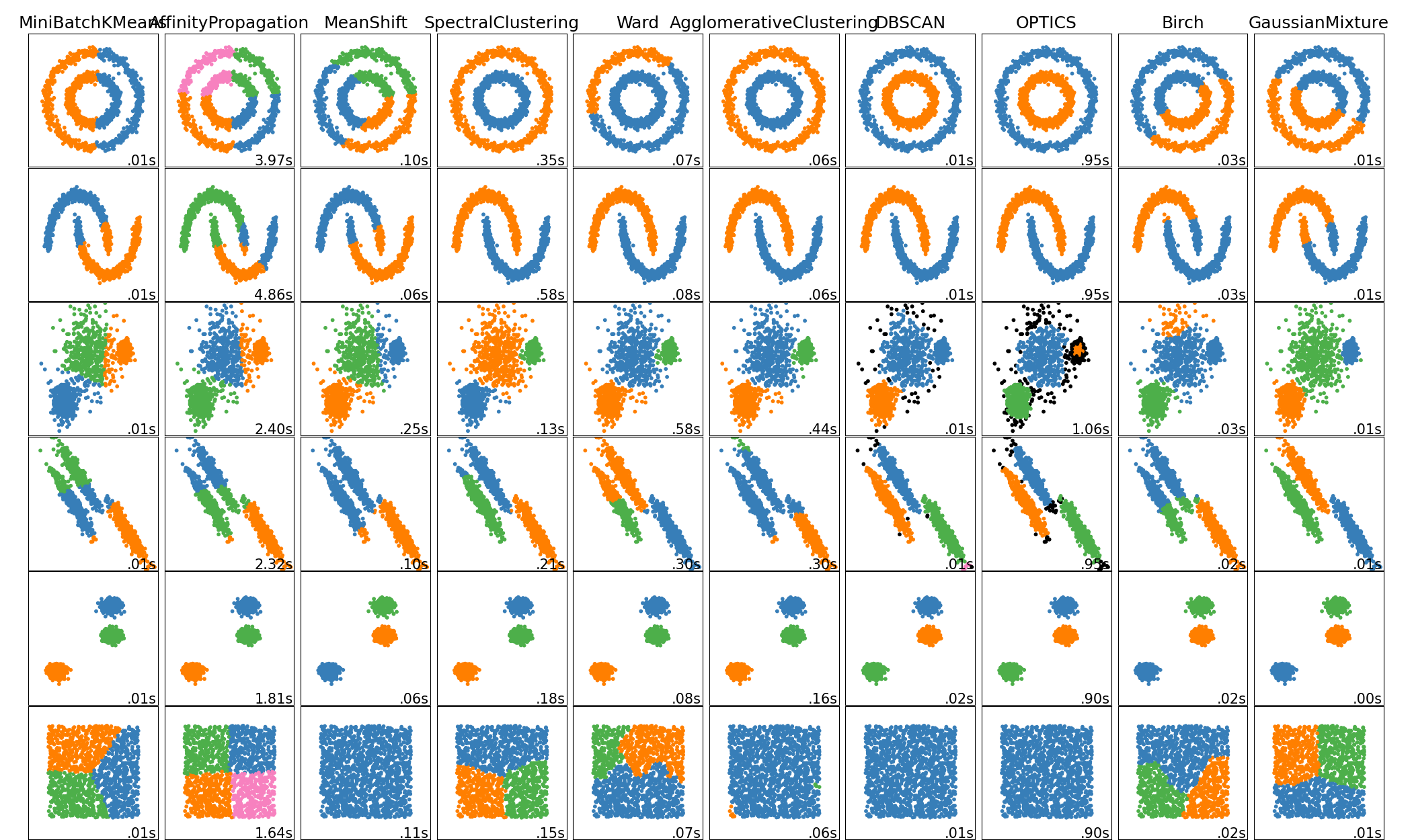# A-Z Clustering.

Original article was published by Bob Rupak Roy on Deep Learning on Medium# Fuzzy Clustering

Fuzzy clustering is a clustering method where data points can belong to more than one group(‘cluster’)

There are 2 ways of clustering we might not know,,

One is ‘Hard Clustering’ and the other was is ‘Soft Clustering / Fuzzy Clustering’

In ‘hard clustering’ each data point can only be 1 cluster but in ‘soft clustering’ or ‘fuzzy clustering’ data point can belong to more than one group.

Fuzzy clustering uses least squares to find the optimal location for any data point. This optimal location maybe in a probability space between two (or more) clusters.

Fuzzy clustering algorithm (FCM) are again divided into 2 areas

1.) Classical fuzzy clustering and 2.) Shape based fuzzy

## 1.) Classical fuzzy Clustering

a.) Fuzzy C-Means algorithm (FCM) . is widely- used algorithm is practically identical to the K-means algorithm. A data point can theoretically belong to one or all groups. Subtype includes Possibilistic C-Means(PCM), Fuzzy Possibilistic C-Means (FPCM) and Possibilistic Fuzzy C-Means (PFCM).

## 2.) Shape-based fuzzy clustering

a.) Circular shaped: circular-shaped (CS) algorithm are what constraints data point to a circular shape. When this algorithm is incorporated into Fuzzy C-Means it’s called CS-FCM

b.) Elliptical Shaped: an algorithm that constrains points to elliptical shapes. Used in the GK algorithm.

c.) Generic shaped. More real life objects are neither circular not elliptical, the generic algorithm allows for clusters of any shape.

Well as of now we will concentrate more with the simple Fuzzy C-Means Clustering.

Steps to perform fuzzy algorithm:

Step 1: Initialize the data points into desired number of clusters randomly.

Step 2: Find out the centroids

Step 3: Find out the distance of each point from centroid

Step 4: Updating membership values

Step 5: Repeat the steps(2–4) until the constant values are obtained for the membership.

## Let’s see how we can perform the fuzzy clustering.

`import numpy as np, numpy.randomimport pandas as pdfrom scipy.spatial import distancek =3p = 2from sklearn import datasetsfrom sklearn.preprocessing import StandardScalerimport pandas as pd#Load datairis = datasets.load_iris()X = iris.data#Standarize featuresscaler = StandardScaler()X1 = scaler.fit_transform(X)#convert to dataframeX1 = pd.DataFrame(X1)X=X1X = pd.DataFrame(X)`

This is our dataset.

`#Print the number of data and dimensionn = len(X)d = len(X.columns)addZeros = np.zeros((n, 1))X = np.append(X, addZeros, axis=1)print("The FCM algorithm: \n")print("The training data: \n", X)print("\nTotal number of data: ",n)print("Total number of features: ",d)print("Total number of Clusters: ",k)#Create an empty array of centersC = np.zeros((k,d+1))#print(C)#Randomly initialize the weight matrixweight = np.random.dirichlet(np.ones(k),size=n)print("\nThe initial weight: \n", np.round(weight,2))for it in range(3): # Total number of iterations#Compute centroidfor j in range(k):denoSum = sum(np.power(weight[:,j],2))sumMM =0for i in range(n):mm = np.multiply(np.power(weight[i,j],p),X[i,:])sumMM +=mmcc = sumMM/denoSumC[j] = np.reshape(cc,d+1)#print("\nUpdating the fuzzy pseudo partition")for i in range(n):denoSumNext = 0for j in range(k):denoSumNext += np.power(1/distance.euclidean(C[j,0:d], X[i,0:d]),1/(p-1))for j in range(k):w = np.power((1/distance.euclidean(C[j,0:d], X[i,0:d])),1/(p-1))/denoSumNextweight[i,j] = wprint("\nThe final weights: \n", np.round(weight,2))`

Its Time to find out our fuzzy clusters.

`for i in range(n):cNumber = np.where(weight[i] == np.amax(weight[i]))X[i,d] = cNumberprint("\nThe data with cluster number: \n", X)`

This is will display the data along with the cluster number else simply click X from variable explorer.

Here we are,,,, the column ‘4’ have the cluster values.

Let me put all the pieces together for you can use it as a template.

`#Fuzzy c means clustering algorithmimport numpy as np, numpy.randomimport pandas as pdfrom scipy.spatial import distancek =3p = 2from sklearn import datasetsfrom sklearn.preprocessing import StandardScalerimport pandas as pd# Load datairis = datasets.load_iris()X = iris.data# Standarize featuresscaler = StandardScaler()X1 = scaler.fit_transform(X)#convert to dataframeX1 = pd.DataFrame(X1)X=X1X = pd.DataFrame(X)#-------------------------------------------------------#Print the number of data and dimension n = len(X)d = len(X.columns)addZeros = np.zeros((n, 1))X = np.append(X, addZeros, axis=1)print("The FCM algorithm: \n")print("The training data: \n", X)print("\nTotal number of data: ",n)print("Total number of features: ",d)print("Total number of Clusters: ",k)#Create an empty array of centersC = np.zeros((k,d+1))#print(C)#Randomly initialize the weight matrixweight = np.random.dirichlet(np.ones(k),size=n)print("\nThe initial weight: \n", np.round(weight,2))for it in range(3): # Total number of iterations    # Compute centroid    for j in range(k):        denoSum = sum(np.power(weight[:,j],2))        sumMM =0        for i in range(n):            mm = np.multiply(np.power(weight[i,j],p),X[i,:])            sumMM +=mm        cc = sumMM/denoSum        C[j] = np.reshape(cc,d+1)    #print("\nUpdating the fuzzy pseudo partition")    for i in range(n):        denoSumNext = 0        for j in range(k):             denoSumNext += np.power(1/distance.euclidean(C[j,0:d], X[i,0:d]),1/(p-1))        for j in range(k):            w = np.power((1/distance.euclidean(C[j,0:d], X[i,0:d])),1/(p-1))/denoSumNext            weight[i,j] = w  print("\nThe final weights: \n", np.round(weight,2))for i in range(n):        cNumber = np.where(weight[i] == np.amax(weight[i]))    X[i,d] = cNumberprint("\nThe data with cluster number: \n", X)#Sum squared error calculationSSE = 0for j in range(k):    for i in range(n):        SSE += np.power(weight[i,j],p)*distance.euclidean(C[j,0:d], X[i,0:d])print("\nSSE: ",np.round(SSE,4))`

Now the some of the widely used applications of Fuzzy Clustering are

Bio-informatics:

One way to use it for pattern recognition technique to analyse gene expression data from microarrays or other. In this case, genes with similar expression/patterns are grouped into the same clusters. Because fuzzy clustering allows genes to belong to more than one cluster. It allows fro the identification of genes that are conditionally co-regulated.

Marketing: customers can be grouped into fuzzy clusters based on their needs, brand choices, psycho-graphic profiles, or other marketing related partitions.

Further it is used for image analysis, medicine, psychology, economics and many other disciplines.

Next another interesting Clustering technique we have, is called ‘Spectral Clustering’